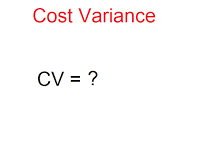"“A clay pot sitting in the sun will always be a clay pot. It has to go through the white heat of the furnace to become porcelain.” - Mildred Struven

## Pages

### PMP®:PMI-ACP®:CAPM® - EVM formula animated

PV - Planned Value. Budget of Planned work
EV - Earned Value. Budget of Completed work.
AC - Actual Cost. Cost spent for Completed work.
BAC - Budget At Completion. Project Budget.

Variances:
Variance means difference. Think of EVM, which means EV is the first value.
In Cost Variance, the first letter and the last letter matches. i.e. Starting and ending with C.In Schedule Variance, the second and the last letter matches. i.e. Second letter and last letter is V.
Indices:
Remember the variances formula and divide instead of subtracting.

At Completion formulae:
EAC - Estimate At Completion. Revised Project Budget.

When original estimate is flawed.
EAC = Cost Spent + estimate for pending work
When the current variance will continue in future also.
EAC=Project Budget divided by Cost Performance Index
When the current variance won't be there in future and pending work will go as per plan.
EAC=Cost Spent+ (Project budget - Earned Value)
When both Cost and Schedule constraint influence the pending work.
Remember the above formula and divide the right side value with CPI*SPI
Variance At Completion: Subtract the other 2 At Completion values in alphabetical order
To Complete Performance Index:
TCPI= Work remaining / Funds remaining

EVM formula worked out wilh Wall construction example

1.Easy to remember the formulas

2.thanks a lot for your very simply of this topic
and I have another hope to explain the EVM of EPC project or Fixed price project
thanks again

3.Thanks Azariah and A.Elhoseiny.

4.Vow Good way to explain.